Wind Tunnel Testing

Tests were conducted in the Aerolab educational wind tunnel at the University of Florida.  The first tests were conducted on March 23 and 24, 2002.  Due to manufacturing problems, the only aluminum blades prepared for the first tests were the straight and 15-20.  In addition, they were only tested at 26, 28, and 30 m/s due to a concern that the motor’s speed controller would overheat due to the additional weight of the aluminum blades.  All polycarbonate blades were tested in the first tests; however, data yielded inconclusive results since the material’s low rigidity allowed deflection during testing.

A second set of wind tunnel tests was conducted, on July 26 and 27, 2002, using only the aluminum blades in order to remove the uncertainty of material flexibility.  The blades tested were the straight, 5-10, 10-15, 15-20, 20-30, 00-15, 10-Forward, and 20-Forward.  Testing was conducted at the speeds of 8, 12, 16, 20, 24, 26, 28, 30, 32, and 34 m/s, which correspond to the advance ratios of 0.04, 0.06, 0.08, 0.10, 0.12, 0.13, 0.14, 0.15, 0.16, and 0.17, respectively.  Because of restrictions caused by testing in a small wind tunnel, measurements were not taken below 8 m/s.

The wind tunnel used was a closed throat model with a cross section of 12”x12”.  To reduce interference of the boundary layer developed in the test section, the propellers were designed to have a diameter of approximately 6 inches.13  Because of the changes in the static pressure inside a closed throat tunnel, a reaction occurs at the propeller causing a higher thrust to be produced at the assumed tunnel velocity than would normally be produced, thus a correction factor is necessary.  The correlation: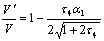(1)

accounts for these effects and was applied to the data in order to negate any bias encountered.13  In this equation, t4 =T/rAV2, a1 =A/C, V′ is the corrected velocity [m/s], V is the freestream velocity [m/s], T is the thrust produced [N], r is the density of the fluid [kg/m3], A is the propeller disk area [m2], and C is the cross-sectional area of the tunnel test section [m2].

The velocity in the test section was controlled by a closed loop feedback control system that regulated the tunnel speed to a specified constant velocity.  Once the tunnel reached the desired speed, the RPM of the propeller motor was then adjusted to 12100 RPM using an optical tachometer, and the system was allowed to arrive at a steady-state condition before data was taken.  Using a computer data acquisition system, the thrust produced by the propeller as well as the current and voltage supplied to the propeller motor was taken every second for 120 seconds.  Statistical analysis of the data from both wind tunnel tests demonstrated that the data set was consistent.

Test Results

For all wind tunnel tests, the efficiency and advance ratio are defined as: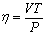(2)

and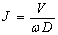(3)

respectively.  In these equations, V is the freestream velocity, T is the thrust produced by the propeller [N], P is the power used [Watts] (found by multiplying the voltage and current consumed by the propellers motor), w is the angular velocity of the propeller [rad/s], and D is the diameter of the propeller [m].  Power losses due to the motor were assumed to be consistent for all blades tested.

Data from all tests performed on the aluminum blades was compiled and then plotted as efficiency vs. advance ratio as shown in Figure 1.  For clarity, only the Graupner, straight, 00-15, 10-15, and 20-Forward blades are shown from the second test.  The straight blade is displayed because it is the basis for comparing the swept designs. The 00-15, 10-15, and 20-Forward blades are shown because they had the largest peak efficiencies and stall margins of all the tested forward swept designs.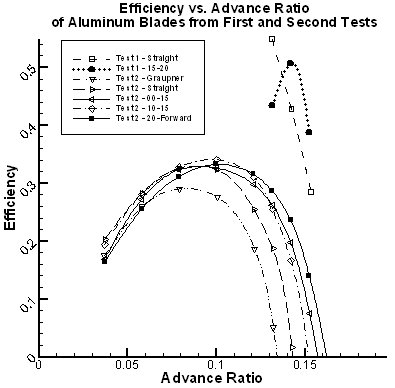Figure 1:  Wind tunnel efficiency vs. advance ratio results

Coefficients of thrust and power are defined as: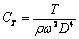(4)

and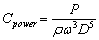(5)

respectively.  In these equations r is the density [kg/m3] and all other variables are as previously defined.  These coefficients are plotted versus advance ratio in Figures 2 and 3 respectively, which together illustrate that the greater thrust produced by the swept blades overcomes the additional power consumed to achieve the higher efficiencies shown.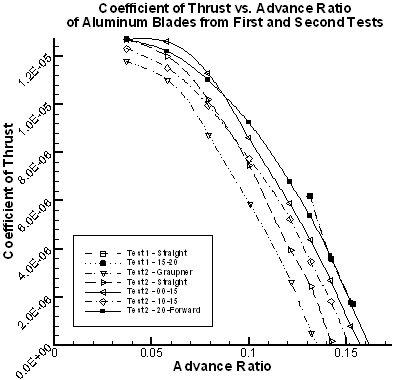Figure 2:  Coefficient of thrust for wind tunnel tests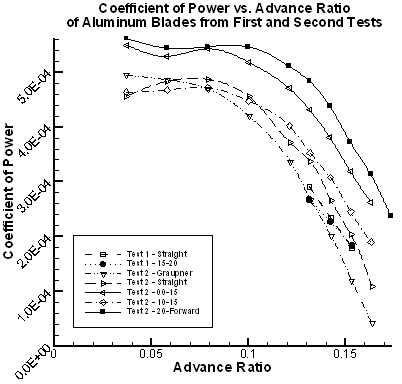Figure 3:  Coefficient of power for wind tunnel tests

Figure 1 also shows a similar trend established in the first test, where the 15-20 blade outperforms the straight.  However, the values from the first test are much higher than those of the second, which indicates that a problem was present in one of the two tests.  It was also realized that a separate measurement should have been taken for the nacelle drag at each speed in order to find the total thrust produced by the propeller.8  These results, however, can still be used as a preliminary guide since the blades will all be affected in the same manner.  Further wind tunnel tests are planned to clarify this uncertainty.